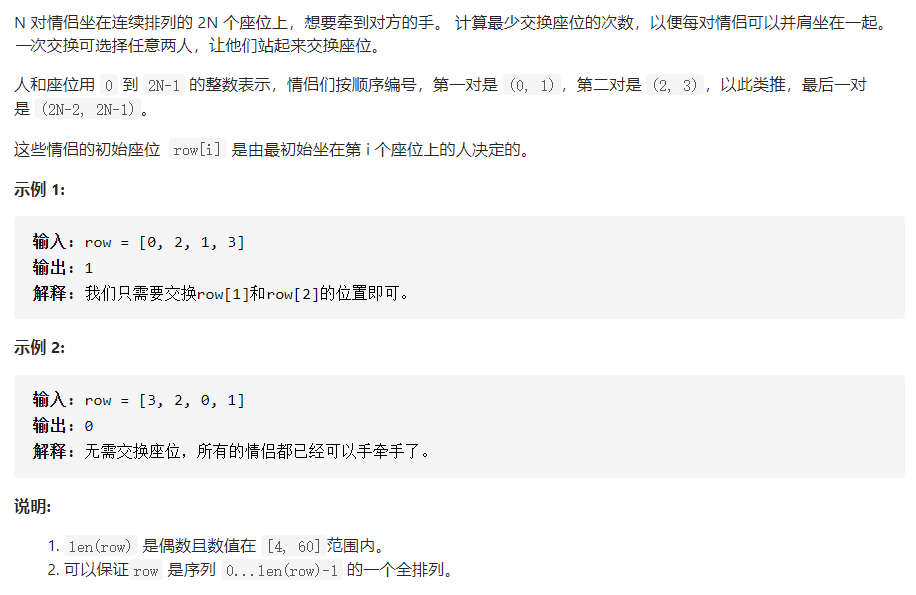# cdcq

## 【力扣765】情侣牵手1.两对情侣必须坐在下标异或为 1 的位置上，也就是说如果一个人在 i ，那么他的对象一定在 i ^ 1

2.对于某个人来说，其余的人只有两种人，他的对象和其他人1 class Solution {
2 public:
3     inline bool checkCouple(int person1, int person2) {
4         return ((person1 ^ person2) == 1);
5     }
6     int minSwapsCouples(vector<int>& row) {
7         int rSize = row.size();
8         int cnt = 0;
9         for(int i = 0; i < rSize; i += 2) {
10             if(!checkCouple(row[i], row[i ^ 1])) {
11                 cnt++;
12                 bool tempMark = false;
13                 for(int j = i + 2; j < rSize; j++) {
14                     if(checkCouple(row[i], row[j]) && checkCouple(row[i ^ 1], row[j ^ 1])) {
15                         tempMark = true;
16                         swap(row[i ^ 1], row[j]);
17                         break;
18                     }
19                     if(checkCouple(row[i ^ 1], row[j]) && checkCouple(row[i], row[j ^ 1])) {
20                         tempMark = true;
21                         swap(row[i], row[j]);
22                         break;
23                     }
24                 }
25                 if(tempMark) {
26                     continue;
27                 }
28                 for(int j = i + 2; j < rSize; j++) {
29                     if(checkCouple(row[i], row[j])) {
30                         swap(row[i ^ 1], row[j]);
31                         break;
32                     }
33                     if(checkCouple(row[i ^ 1], row[j])) {
34                         swap(row[i], row[j]);
35                         break;
36                     }
37                 }
38             }
39         }
40         return cnt;
41     }
42 };
View Code

posted on 2021-02-14 13:37  cdcq  阅读(44)  评论(0编辑  收藏